# The net magnetic field at the center

PhysicsTest
Homework Statement:
Four, long, parallel power lines each carry 100-A currents. A cross-sectional diagram of these lines is a square, 20.0 cm on each side. For each of the three cases shown in , calculate the magnetic field at the center of the square.
Relevant Equations:
##B = \frac {\mu_0 I} {2a}##
The current direction is as followsI think so much and do the right hand rule i get 0 at the center, but not sure why the answer is non zero. I have shown the directions of the magnetic fields, i have not shown the magnitudes of equal length but they all are equal. Why the answer is non zero, what mistake i am doing?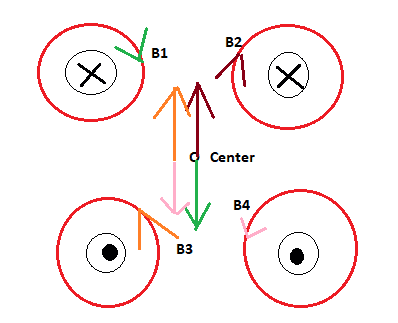Homework Helper
I see only one case ? Mixup of answers ?

PhysicsTest
I see only one case ? Mixup of answers ?
The first two I could solve, this is the 3rd one which I am struggling to get answer.

Gordianus
May I suggest drawing the arrows again?

•BvU
PhysicsTest
May I suggest drawing the arrows again?
I end up getting below the same,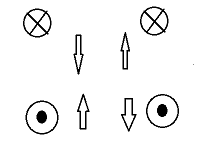Gordianus
No, try again.

PhysicsTest
No, try again.
Ok, chances are there of a below diagram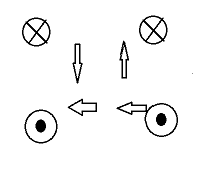But a very tough one to know where it will be left. Am i correct here?

Gordianus
Try drawing the B-lines of each wire

PhysicsTest
Try drawing the B-lines of each wire
The B lines are like this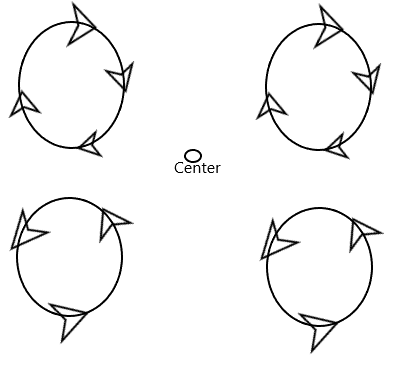Everything seems to be left.

Gordianus
Good! Now extend the lines to the center and draw the arrows again.

•hutchphd
PhysicsTest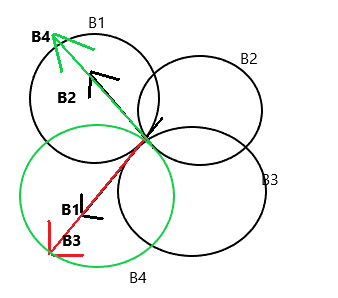Surely the tougher than i expected. Hope it is correct.

Gordianus
Victory!

•PhysicsTest
PhysicsTest
Thank you very much for support.